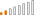cancel
Showing results for
Did you mean:Continued Contributor

## Case statement in power query but with a greater than operator

Trying to implement the following in power query, I tried adding a conditional column but don't know how to get it to work with a greater than operator.

case

when column1 >= 20

and column1 <= 100

and column2 = 'N' then 1

else 0

end as column

1 ACCEPTED SOLUTIONContinued Contributor

I got it to work:  Add column -> custom column, use the following formula:

if [column1] >= 20 and [column1] <= 100 and [column2] = "N" then 1 else 0

if column1 is a text datatype then you need to surround the value with double quotesContinued Contributor

I got it to work:  Add column -> custom column, use the following formula:

if [column1] >= 20 and [column1] <= 100 and [column2] = "N" then 1 else 0

if column1 is a text datatype then you need to surround the value with double quotes